Technical Information Site of Power Supply Design

• Basic Knowledge
• AC/DC
• Design Example of Isolated Quasi-Resonant Converters Using SiC MOSFET
• Selecting Critical Components: Output Capacitors, Output Setting and Control Components

2019.03.07 AC/DC

# Selecting Critical Components: Output Capacitors, Output Setting and Control Components

Design Example of Isolated Quasi-Resonant Converters Using SiC MOSFET

In succession to the previous article, we select components related to the secondary side (output).

Output Capacitors：Cout1, Cout2

Let's review the action of the output capacitor. When the MOSFET is turned on, the output diode DN1 is in the off state. At this time, a current is supplied to the load from the output capacitor. When the MOSFET is turned off, the output diode DN1 is in the on state, and at this time the output capacitor is charged, and moreover the load current is supplied.

The output capacitor is determined from the peak-to-peak ripple voltage (ΔVpp) that can be tolerated by the output load and the ripple current. We begin by determining the capacitor impedance Z_C.

If ΔVpp=200 mV, then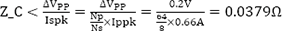*At 60 kHz（fsw min）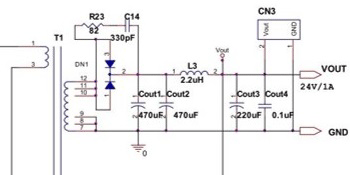The impedance of an electrolytic capacitor (low-impedance device) for general switching power supplies is defined as the value at 100 kHz, and so this is converted into the 100 kHz value.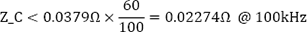Next, the ripple current Is(rms) is determined. Is is found using the following equation.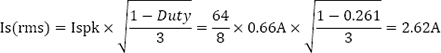Next, the capacitor voltage rating is assumed to be about twice the output voltage.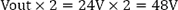A device with a voltage rating of 50 V or higher is selected.

An electrolytic capacitor is selected that matches the conditions of an impedance at or below the calculated value, a ripple current rating at or above the calculated value, and a voltage rating of 50 V or above. In the circuit example, low impedance type capacitors, 50 V/470 μF × 2 (in parallel) for switching power supplies, are used.

The actual ripple voltage and ripple current should always be confirmed using actual equipment.

Output Voltage Setting Resistors：R25, R26, R28, U3

The following equation is used to set the output voltage. U3 is a TL431 type shunt regulator that produces a reference voltage Vref used to set the output voltage. Vref is set to 2.495 V.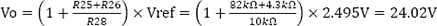In actuality, calculate backwards from the output voltage.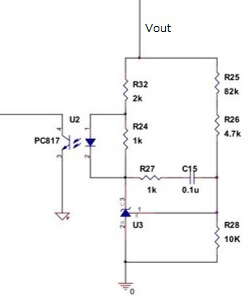Feedback Signal Adjustment Components：R24, R27, R32, C15, U2

In this circuit example, Vout is adjusted using the circuit on the right in order to stabilize the output voltage Vout, and the result is fed back to the FB pin of the power supply IC through a photocoupler for isolation.

R27 and C15 form a phase compensation circuit. Actual equipment should be used to make adjustments while checking the response, with R27 set to 1 kΩ to 30 kΩ and C15 at approximately 0.1 μF.

R32 is a current limiter resistor of the photocoupler U2. It should be adjusted in the range 300 to 2 kΩ.

R24 is a cathode current setting resistor for the shunt regulator U3. The TL431 U3 requires that 1 mA be secured, and so R24 is set to Vf/1 mA = 1 kΩ for the photocoupler.

#### Key Points:

・An output capacitor is selected using the peak-to-peak ripple voltage (ΔVpp) that can be tolerated by the output load and the ripple current.

・The output voltage setting resistor can be calculated from an equation given by the data sheet.

・Feedback signal adjustment components should conform to values given on the data sheet.